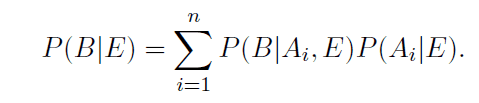# The law of total probability with extra conditioning

• B
red65
TL;DR Summary
the proof of a theorem
Hello, I am studying probability and came across this theorem, it's the law of total probability with extra conditioning, I tried to work out a proof but couldn't ,does anyone know the proof for this :thanks!

Homework Helper
Gold Member
1) If you are looking for a proof, you should be very careful about the exact statement. There is more to that statement, right? Don't the ##A_i## need to be a partitioning?
2) If all probabilities are conditional on ##E##, isn't that just another probability where ##E## is the universe and does not need to be included in the notation?

•PeroK
Homework Helper
Gold Member
2022 Award
TL;DR Summary: the proof of a theorem

Hello, I am studying probability and came across this theorem, it's the law of total probability with extra conditioning, I tried to work out a proof but couldn't ,does anyone know the proof for this :
View attachment 319864
thanks!
That's just the usual equation with a restriction to ##E## as the universal set or sample space. Given the proviso, as above, that the ##A_i## (when restricted to ##E##) partition ##E##.

•FactChecker